Solving Pair of Linear Inequalities

Chapter 5 Class 11 Linear Inequalities
Serial order wise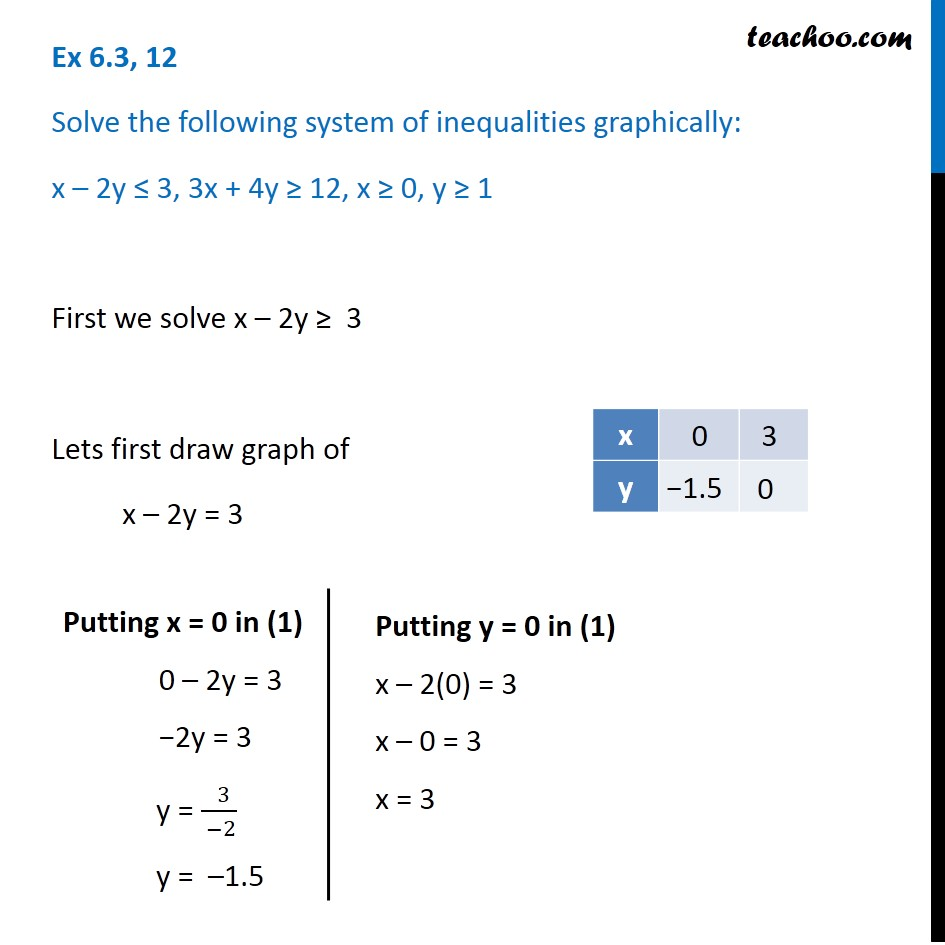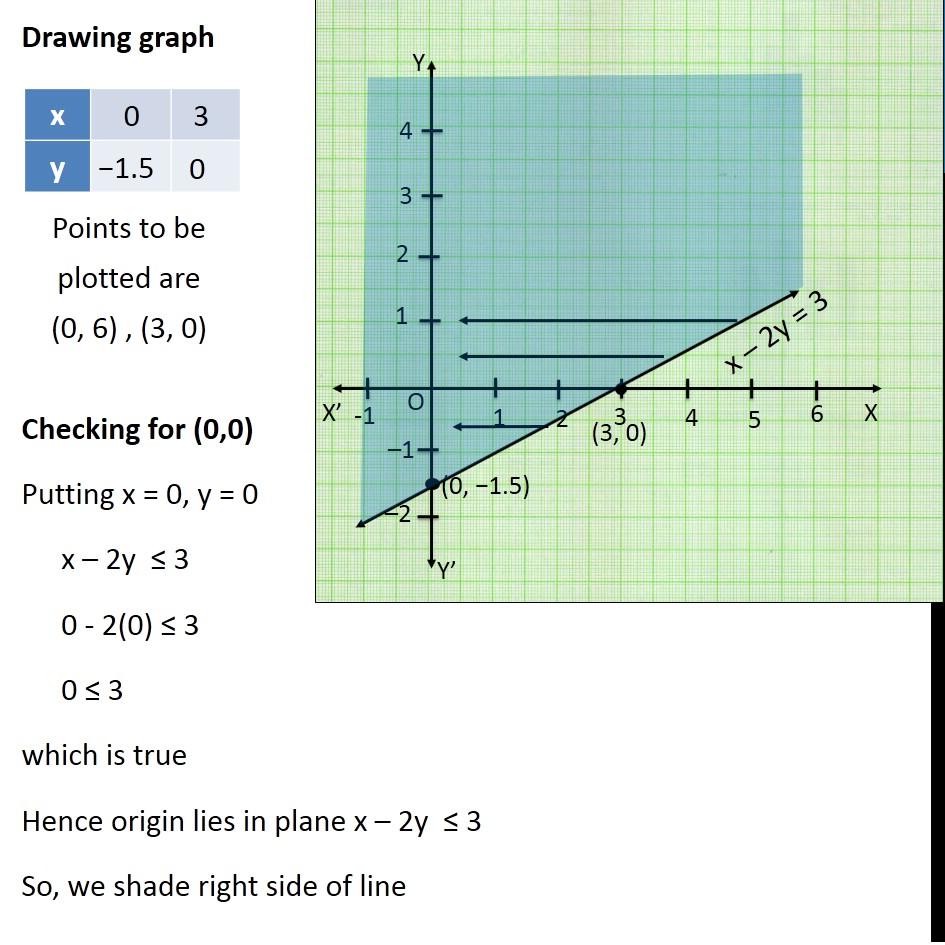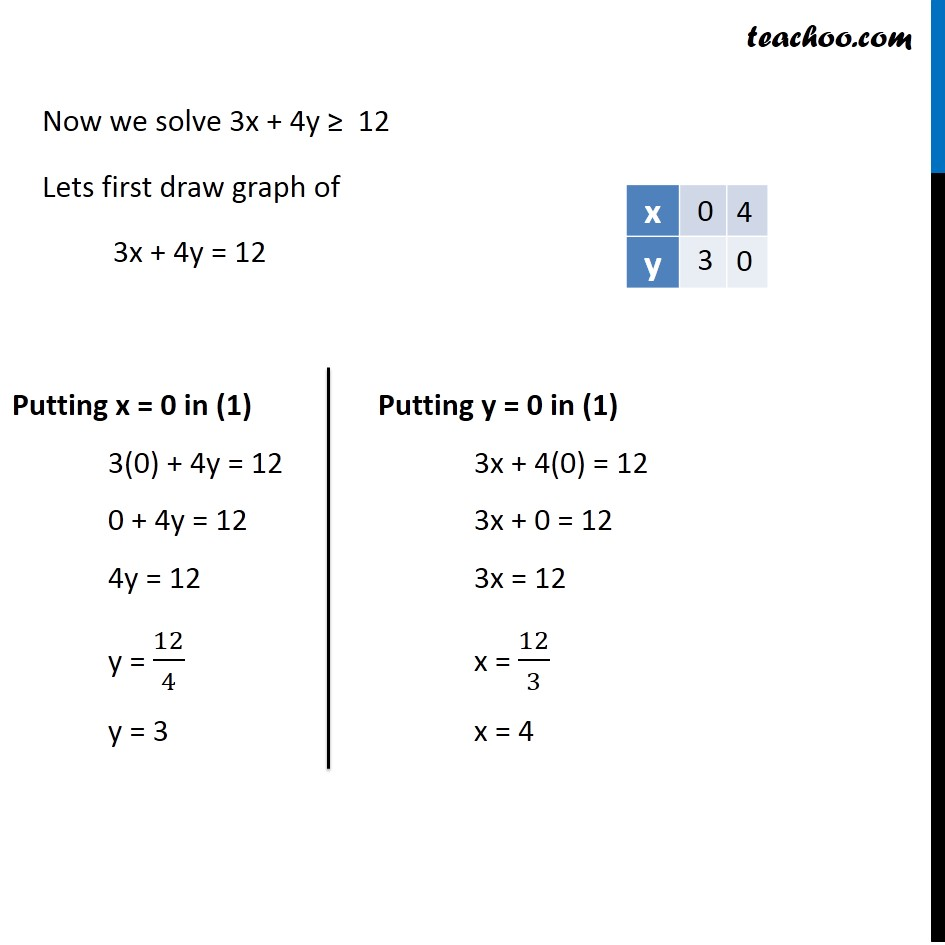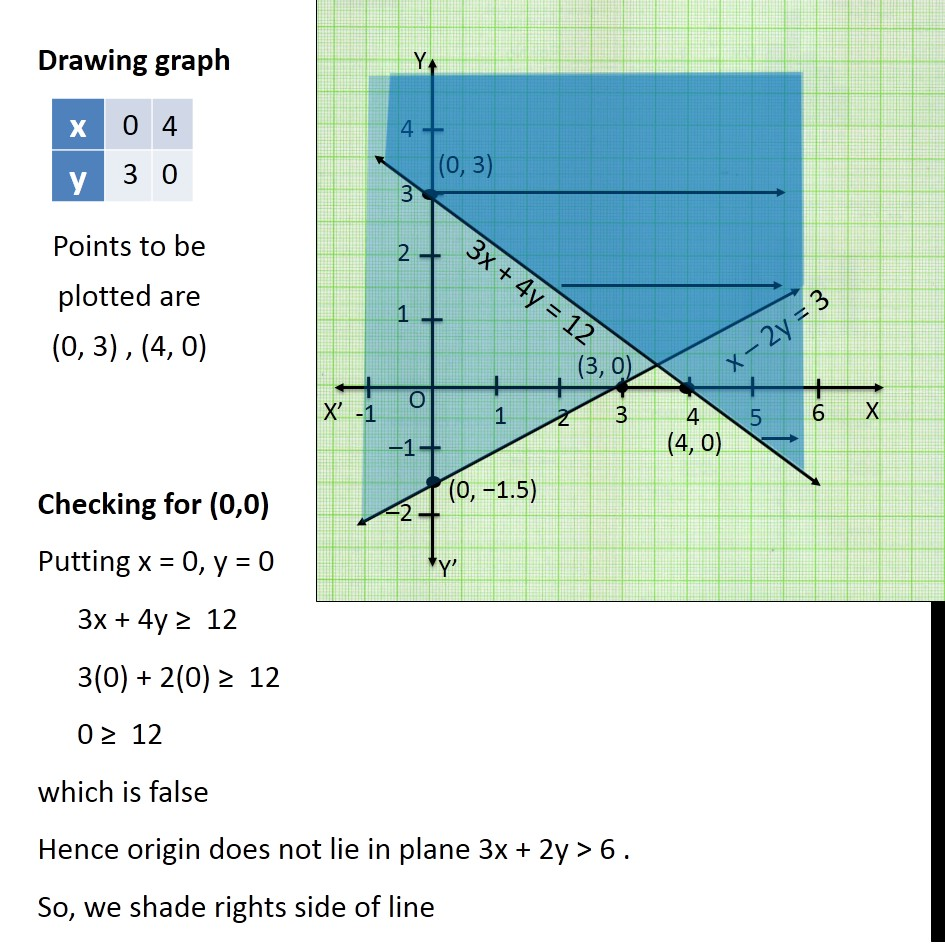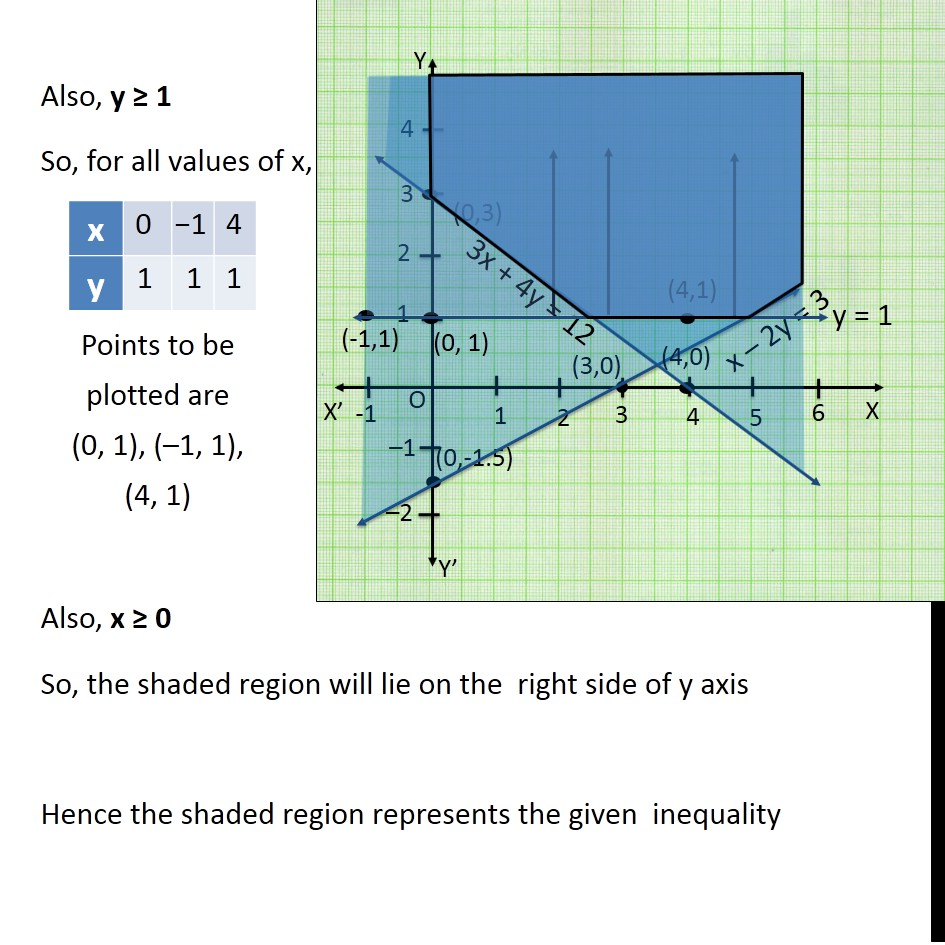Learn in your speed, with individual attention - Teachoo Maths 1-on-1 Class

### Transcript

Question 12 Solve the following system of inequalities graphically: x – 2y ≤ 3, 3x + 4y ≥ 12, x ≥ 0, y ≥ 1 First we solve x – 2y ≥ 3 Lets first draw graph of x – 2y = 3 Putting x = 0 in (1) 0 – 2y = 3 −2y = 3 y = ( 3)/( −2) y = –1.5 Putting y = 0 in (1) x – 2(0) = 3 x – 0 = 3 x = 3 Drawing graph Checking for (0,0) Putting x = 0, y = 0 x – 2y ≤ 3 0 - 2(0) ≤ 3 0 ≤ 3 which is true Hence origin lies in plane x – 2y ≤ 3 So, we shade right side of line Points to be plotted are (0, 6) , (3, 0) Now we solve 3x + 4y ≥ 12 Lets first draw graph of 3x + 4y = 12 Putting x = 0 in (1) 3(0) + 4y = 12 0 + 4y = 12 4y = 12 y = 12/4 y = 3 Putting y = 0 in (1) 3x + 4(0) = 12 3x + 0 = 12 3x = 12 x = 12/3 x = 4 Points to be plotted are (0, 3) , (4, 0) Drawing graph Checking for (0,0) Putting x = 0, y = 0 3x + 4y ≥ 12 3(0) + 2(0) ≥ 12 0 ≥ 12 which is false Hence origin does not lie in plane 3x + 2y > 6 . So, we shade rights side of line Points to be plotted are (0, 1), (–1, 1), (4, 1) Also, y ≥ 1 So, for all values of x, y = 2 Also, x ≥ 0 So, the shaded region will lie on the right side of y axis Hence the shaded region represents the given inequality